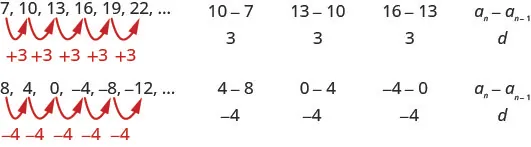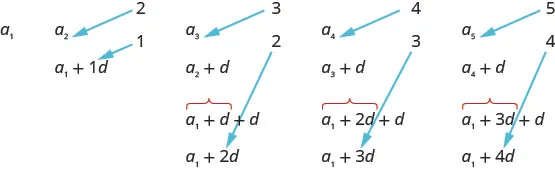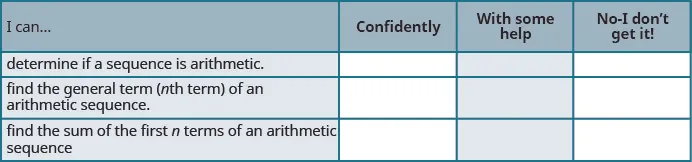Intermediate Algebra 2e

# 12.2Arithmetic Sequences

Intermediate Algebra 2e12.2 Arithmetic Sequences

### Learning Objectives

By the end of this section, you will be able to:

• Determine if a sequence is arithmetic
• Find the general term ($nn$th term) of an arithmetic sequence
• Find the sum of the first $nn$ terms of an arithmetic sequence

### Be Prepared 12.4

Before you get started, take this readiness quiz.

Evaluate $4n−14n−1$ for the integers 1, 2, 3, and 4.
If you missed this problem, review Example 1.6.

### Be Prepared 12.5

Solve the system of equations: ${x+y=73x+4y=23.{x+y=73x+4y=23.$
If you missed this problem, review Example 4.9.

### Be Prepared 12.6

If $f(n)=n2(3n+5),f(n)=n2(3n+5),$ find $f(1)+f(20).f(1)+f(20).$
If you missed this problem, review Example 3.49.

### Determine if a Sequence is Arithmetic

The last section introduced sequences and now we will look at two specific types of sequences that each have special properties. In this section we will look at arithmetic sequences and in the next section, geometric sequences.

An arithmetic sequence is a sequence where the difference between consecutive terms is constant. The difference between consecutive terms in an arithmetic sequence, $an−an−1,an−an−1,$ is d, the common difference, for n greater than or equal to two.

### Arithmetic Sequence

An arithmetic sequence is a sequence where the difference between consecutive terms is always the same.

The difference between consecutive terms, $an−an−1,an−an−1,$ is d, the common difference, for n greater than or equal to two.In each of these sequences, the difference between consecutive terms is constant, and so the sequence is arithmetic.

### Example 12.13

Determine if each sequence is arithmetic. If so, indicate the common difference.

$5,9,13,17,21,25,…5,9,13,17,21,25,…$

$4,9,12,17,20,25,…4,9,12,17,20,25,…$

$10,3,−4,−11,−18,−25,…10,3,−4,−11,−18,−25,…$

### Try It 12.25

Determine if each sequence is arithmetic. If so, indicate the common difference.

$9,20,31,42,53,64,…9,20,31,42,53,64,…$ $12,6,0,−6,−12,−18,…12,6,0,−6,−12,−18,…$ $7,1,10,4,13,7,…7,1,10,4,13,7,…$

### Try It 12.26

Determine if each sequence is arithmetic. If so, indicate the common difference.

$−4,4,2,10,8,16,…−4,4,2,10,8,16,…$ $−3,−1,1,3,5,7,…−3,−1,1,3,5,7,…$ $7,2,−3,−8,−13,−18,…7,2,−3,−8,−13,−18,…$

If we know the first term, $a1,a1,$ and the common difference, d, we can list a finite number of terms of the sequence.

### Example 12.14

Write the first five terms of the sequence where the first term is 5 and the common difference is $d=−6.d=−6.$

### Try It 12.27

Write the first five terms of the sequence where the first term is 7 and the common difference is $d=−4.d=−4.$

### Try It 12.28

Write the first five terms of the sequence where the first term is 11 and the common difference is $d=−8.d=−8.$

### Find the General Term (nth Term) of an Arithmetic Sequence

Just as we found a formula for the general term of a sequence, we can also find a formula for the general term of an arithmetic sequence.

Let’s write the first few terms of a sequence where the first term is $a1a1$ and the common difference is d. We will then look for a pattern.

As we look for a pattern we see that each term starts with $a1a1$.The first term adds 0d to the $a1a1$, the second term adds 1d, the third term adds 2d, the fourth term adds 3d, and the fifth term adds 4d. The number of ds that were added to $a1a1$ is one less than the number of the term. This leads us to the following

$an=a1+(n−1)dan=a1+(n−1)d$

### General Term (nth term) of an Arithmetic Sequence

The general term of an arithmetic sequence with first term $a1a1$ and the common difference d is

$an=a1+(n−1)dan=a1+(n−1)d$

We will use this formula in the next example to find the 15th term of a sequence.

### Example 12.15

Find the fifteenth term of a sequence where the first term is 3 and the common difference is 6.

### Try It 12.29

Find the twenty-seventh term of a sequence where the first term is 7 and the common difference is 9.

### Try It 12.30

Find the eighteenth term of a sequence where the first term is 13 and the common difference is $−7−7$.

Sometimes we do not know the first term and we must use other given information to find it before we find the requested term.

### Example 12.16

Find the twelfth term of a sequence where the seventh term is 10 and the common difference is $−2−2$. Give the formula for the general term.

### Try It 12.31

Find the eleventh term of a sequence where the ninth term is 8 and the common difference is $−3.−3.$ Give the formula for the general term.

### Try It 12.32

Find the nineteenth term of a sequence where the fifth term is 1 and the common difference is $−4.−4.$ Give the formula for the general term.

Sometimes the information given leads us to two equations in two unknowns. We then use our methods for solving systems of equations to find the values needed.

### Example 12.17

Find the first term and common difference of a sequence where the fifth term is 19 and the eleventh term is 37. Give the formula for the general term.

### Try It 12.33

Find the first term and common difference of a sequence where the fourth term is 17 and the thirteenth term is 53. Give the formula for the general term.

### Try It 12.34

Find the first term and common difference of a sequence where the third term is 2 and the twelfth term is $−25.−25.$ Give the formula for the general term.

### Find the Sum of the First n Terms of an Arithmetic Sequence

As with the general sequences, it is often useful to find the sum of an arithmetic sequence. The sum, $Sn,Sn,$ of the first $nn$ terms of any arithmetic sequence is written as $Sn=a1+a2+a3+...+an.Sn=a1+a2+a3+...+an.$ To find the sum by merely adding all the terms can be tedious. So we can also develop a formula to find the sum of a sequence using the first and last term of the sequence.

We can develop this new formula by first writing the sum by starting with the first term, $a1,a1,$ and keep adding a d to get the next term as:

$Sn=a1+(a1+d)+(a1+2d)+…+an.Sn=a1+(a1+d)+(a1+2d)+…+an.$

We can also reverse the order of the terms and write the sum by starting with $anan$ and keep subtracting d to get the next term as

$Sn=an+(an–d)+(an–2d)+…+a1.Sn=an+(an–d)+(an–2d)+…+a1.$

If we add these two expressions for the sum of the first n terms of an arithmetic sequence, we can derive a formula for the sum of the first n terms of any arithmetic series.

$Sn=a1+(a1+d)+(a1+2d)+…+an+Sn=an+(an−d)+(an−2d)+…+a1_________________________________________________________2Sn=(a1+an)+(a1+an)+(a1+an)+…+(a1+an)Sn=a1+(a1+d)+(a1+2d)+…+an+Sn=an+(an−d)+(an−2d)+…+a1_________________________________________________________2Sn=(a1+an)+(a1+an)+(a1+an)+…+(a1+an)$

Because there are n sums of $(a1+an)(a1+an)$ on the right side of the equation, we rewrite the right side as $n(a1+an).n(a1+an).$

$2Sn=n(a1+an)2Sn=n(a1+an)$

We divide by two to solve for $Sn.Sn.$

$Sn=n2(a1+an)Sn=n2(a1+an)$

This gives us a general formula for the sum of the first n terms of an arithmetic sequence.

### Sum of the First n Terms of an Arithmetic Sequence

The sum, $Sn,Sn,$ of the first n terms of an arithmetic sequence is

$Sn=n2(a1+an)Sn=n2(a1+an)$

where $a1a1$ is the first term and $anan$ is the nth term.

We apply this formula in the next example where the first few terms of the sequence are given.

### Example 12.18

Find the sum of the first 30 terms of the arithmetic sequence: 8, 13, 18, 23, 28, …

### Try It 12.35

Find the sum of the first 30 terms of the arithmetic sequence: 5, 9, 13, 17, 21, …

### Try It 12.36

Find the sum of the first 30 terms of the arithmetic sequence: 7, 10, 13, 16, 19, …

In the next example, we are given the general term for the sequence and are asked to find the sum of the first 50 terms.

### Example 12.19

Find the sum of the first 50 terms of the arithmetic sequence whose general term is $an=3n−4.an=3n−4.$

### Try It 12.37

Find the sum of the first 50 terms of the arithmetic sequence whose general term is $an=2n−5.an=2n−5.$

### Try It 12.38

Find the sum of the first 50 terms of the arithmetic sequence whose general term is $an=4n+3.an=4n+3.$

In the next example we are given the sum in summation notation. To add all the terms would be tedious, so we extract the information needed to use the formula to find the sum of the first n terms.

### Example 12.20

Find the sum: $∑i=125(4i+7).∑i=125(4i+7).$

### Try It 12.39

Find the sum: $∑i=130(6i−4).∑i=130(6i−4).$

### Try It 12.40

Find the sum: $∑i=135(5i−3).∑i=135(5i−3).$

### Media

Access these online resources for additional instruction and practice with arithmetic sequences

### Section 12.2 Exercises

#### Practice Makes Perfect

Determine if a Sequence is Arithmetic

In the following exercises, determine if each sequence is arithmetic, and if so, indicate the common difference.

77.

$4 , 12 , 20 , 28 , 36 , 44 , … 4 , 12 , 20 , 28 , 36 , 44 , …$

78.

$−7 , −2 , 3 , 8 , 13 , 18 , … −7 , −2 , 3 , 8 , 13 , 18 , …$

79.

$−15 , −16 , 3 , 12 , 21 , 30 , … −15 , −16 , 3 , 12 , 21 , 30 , …$

80.

$11 , 5 , −1 , −7 − 13 , −19 , … 11 , 5 , −1 , −7 − 13 , −19 , …$

81.

$8 , 5 , 2 , −1 , −4 , −7 , … 8 , 5 , 2 , −1 , −4 , −7 , …$

82.

$15 , 5 , −5 , −15 , −25 , −35 , … 15 , 5 , −5 , −15 , −25 , −35 , …$

In the following exercises, write the first five terms of each sequence with the given first term and common difference.

83.

$a1=11a1=11$ and $d=7d=7$

84.

$a1=18a1=18$ and $d=9d=9$

85.

$a1=−7a1=−7$ and $d=4d=4$

86.

$a1=−8a1=−8$ and $d=5d=5$

87.

$a1=14a1=14$ and $d=−9d=−9$

88.

$a1=−3a1=−3$ and $d=−3d=−3$

Find the General Term (nth Term) of an Arithmetic Sequence

In the following exercises, find the term described using the information provided.

89.

Find the twenty-first term of a sequence where the first term is three and the common difference is eight.

90.

Find the twenty-third term of a sequence where the first term is six and the common difference is four.

91.

Find the thirtieth term of a sequence where the first term is $−14−14$ and the common difference is five.

92.

Find the fortieth term of a sequence where the first term is $−19−19$ and the common difference is seven.

93.

Find the sixteenth term of a sequence where the first term is 11 and the common difference is $−6.−6.$

94.

Find the fourteenth term of a sequence where the first term is eight and the common difference is $−3.−3.$

95.

Find the twentieth term of a sequence where the fifth term is $−4−4$ and the common difference is $−2.−2.$ Give the formula for the general term.

96.

Find the thirteenth term of a sequence where the sixth term is $−1−1$ and the common difference is $−4.−4.$ Give the formula for the general term.

97.

Find the eleventh term of a sequence where the third term is 19 and the common difference is five. Give the formula for the general term.

98.

Find the fifteenth term of a sequence where the tenth term is 17 and the common difference is seven. Give the formula for the general term.

99.

Find the eighth term of a sequence where the seventh term is $−8−8$ and the common difference is $−5.−5.$ Give the formula for the general term.

100.

Find the fifteenth term of a sequence where the tenth term is $−11−11$ and the common difference is $−3.−3.$ Give the formula for the general term.

In the following exercises, find the first term and common difference of the sequence with the given terms. Give the formula for the general term.

101.

The second term is 14 and the thirteenth term is 47.

102.

The third term is 18 and the fourteenth term is 73.

103.

The second term is 13 and the tenth term is $−51.−51.$

104.

The third term is four and the tenth term is $−38−38$.

105.

The fourth term is $−6−6$ and the fifteenth term is 27.

106.

The third term is $−13−13$ and the seventeenth term is 15.

Find the Sum of the First n Terms of an Arithmetic Sequence

In the following exercises, find the sum of the first 30 terms of each arithmetic sequence.

107.

$11 , 14 , 17 , 20 , 23 , … 11 , 14 , 17 , 20 , 23 , …$

108.

$12 , 18 , 24 , 30 , 36 , … 12 , 18 , 24 , 30 , 36 , …$

109.

$8 , 5 , 2 , −1 , −4 , … 8 , 5 , 2 , −1 , −4 , …$

110.

$16 , 10 , 4 , −2 , −8 , … 16 , 10 , 4 , −2 , −8 , …$

111.

$−17 , −15 , −13 , −11 , −9 , … −17 , −15 , −13 , −11 , −9 , …$

112.

$−15 , −12 , −9 , −6 , −3 , … −15 , −12 , −9 , −6 , −3 , …$

In the following exercises, find the sum of the first 50 terms of the arithmetic sequence whose general term is given.

113.

$a n = 5 n − 1 a n = 5 n − 1$

114.

$a n = 2 n + 7 a n = 2 n + 7$

115.

$a n = −3 n + 5 a n = −3 n + 5$

116.

$a n = −4 n + 3 a n = −4 n + 3$

In the following exercises, find each sum.

117.

$∑ i = 1 40 ( 8 i − 7 ) ∑ i = 1 40 ( 8 i − 7 )$

118.

$∑ i = 1 45 ( 7 i − 5 ) ∑ i = 1 45 ( 7 i − 5 )$

119.

$∑ i = 1 50 ( 3 i + 6 ) ∑ i = 1 50 ( 3 i + 6 )$

120.

$∑ i = 1 25 ( 4 i + 3 ) ∑ i = 1 25 ( 4 i + 3 )$

121.

$∑ i = 1 35 ( −6 i − 2 ) ∑ i = 1 35 ( −6 i − 2 )$

122.

$∑ i = 1 30 ( −5 i + 1 ) ∑ i = 1 30 ( −5 i + 1 )$

#### Writing Exercises

123.

In your own words, explain how to determine whether a sequence is arithmetic.

124.

In your own words, explain how the first two terms are used to find the tenth term. Show an example to illustrate your explanation.

125.

In your own words, explain how to find the general term of an arithmetic sequence.

126.

In your own words, explain how to find the sum of the first $nn$ terms of an arithmetic sequence without adding all the terms.

#### Self Check

After completing the exercises, use this checklist to evaluate your mastery of the objectives of this section.After reviewing this checklist, what will you do to become confident for all objectives?

Order a print copy

As an Amazon Associate we earn from qualifying purchases.